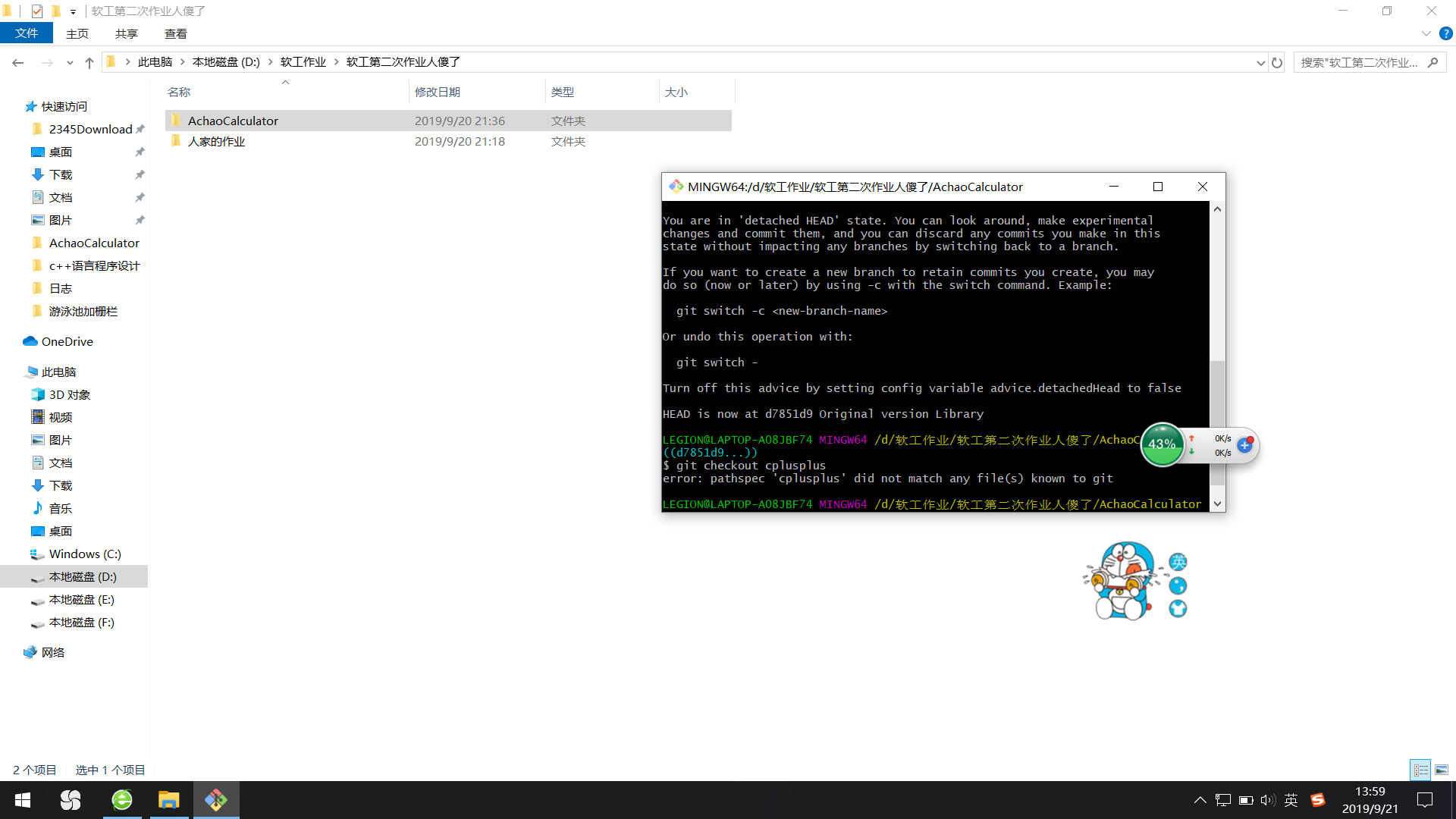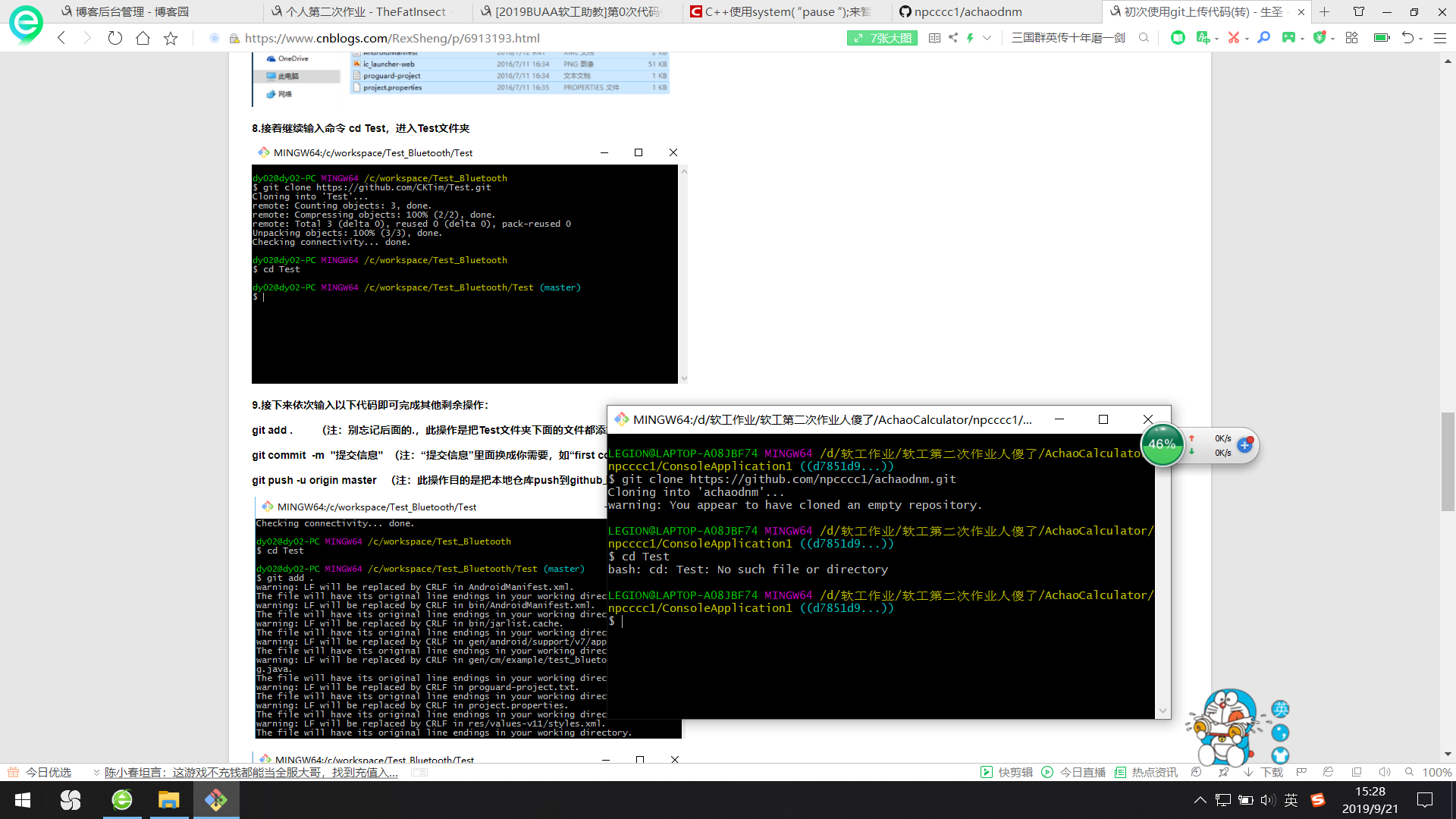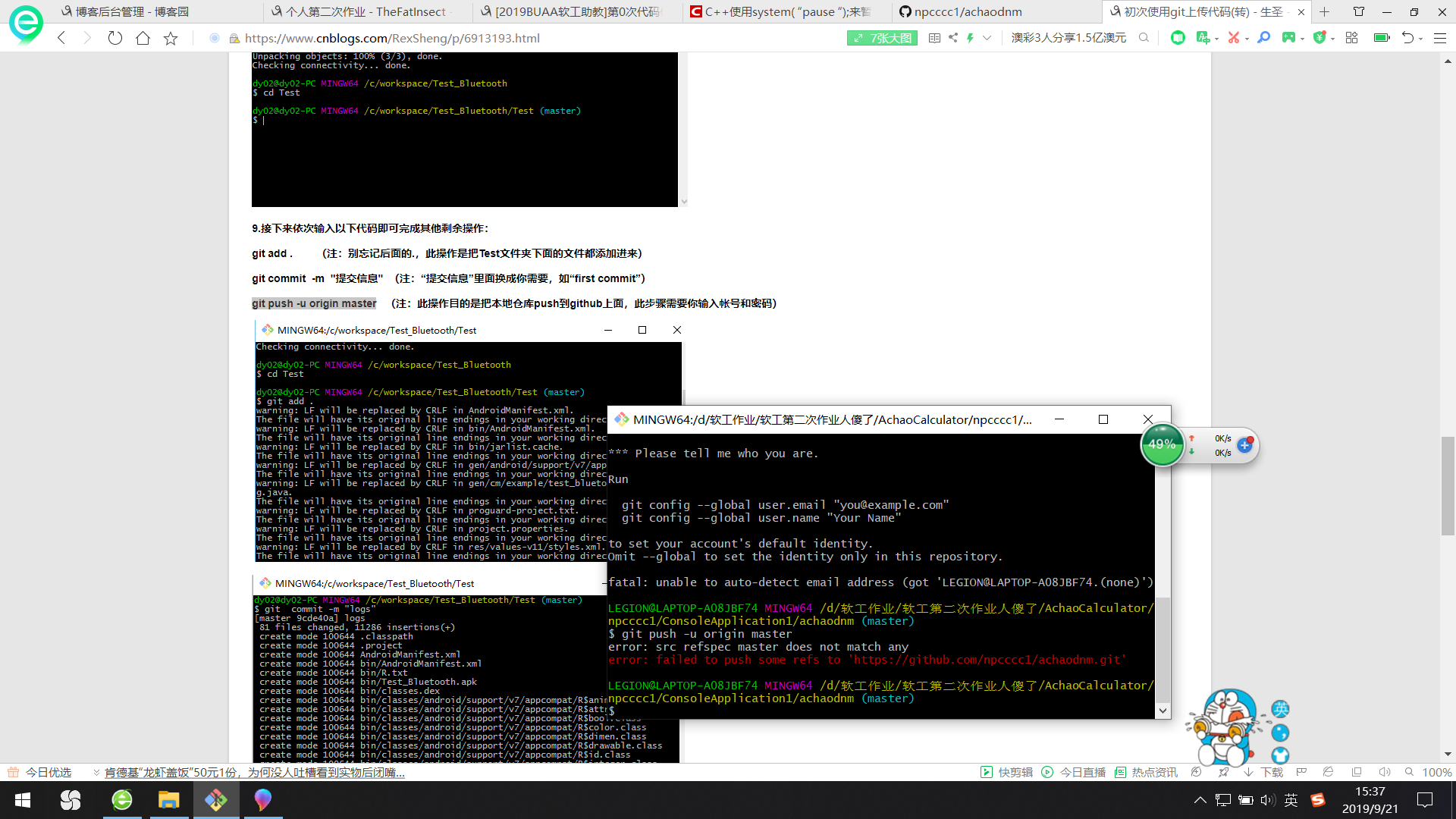git地址 https://github.com/npcccc1/achaodnm.git git用户名 npcccc1 博客链接 https://www.cnblogs.com/npc1158947015/ 学号后5位 92324 作业链接 https://edu.cnblogs.com/campus/xnsy/Autumn2019SoftwareEngineeringFoundation/homework/7590

# 一、环境配置过程# 二、代码思路

13 + 17 - 1 = 29
11 * 15 - 5 = 160
3 + 10 + 4 - 16 = 1
15÷5 + 3 - 2 = 4

#include "pch.h"                    //头文件
#include <iostream>
#include <string.h>
#include <stdlib.h>
#include <time.h>
using namespace std;


char Operator()
{
int i;
srand(time(NULL));            //需要生成随机的运算符与随机数
i = rand() % 4 + 1;           //就先设出这两个函数
switch (i)
{
case 1: return '+';
case 2: return '-';
case 3: return '*';
case 4: return '/';
}
}

int Random()
{
int x;
srand(time(NULL));           //看看是否为伪随机数
x = rand() % 100 + 1;
return x;
}


先把主函数列上，方便之后的函数解说

int main()
{
int a,b,c,d,judge,n,x;
char op1,op2,op3;
cout << "enter the number of calculations that you want to produce:"<<endl;
cin >> n;                    //问要几个四则运算
for (x = 1; x <= n; x++)
{
judge = rand() % 3 + 1;
if (judge = 1)            //judge为运算符个数，1时再加1或2
{
judge = judge + rand() % 2 + 1;
}
if (judge == 2)
{
a = Random();
b = Random();
c = Random();
op1 = Operator();
op2 = Operator();
Calculation(op1, a, b);     //把两随机数与符号输出
Calculation(op2, c);       //用到函数重载
cout << endl;
}
if (judge == 3)
{                                   //三个运算符需要四个随机数构成式子
a = Random();
b = Random();
c = Random();
d = Random();
op1 = Operator();
op2 = Operator();
op3 = Operator();
Calculation(op1, a, b);
Calculation(op2, c);
Calculation(op3, d);
cout << endl;
}
}
}


函数Calculation与Realmark（判断除法运算结果是否为小数）与全局变量int temporary：

一开始想的是只写一个Calculation(op1, a, b)函数，在txt文件中把相邻的两个相同的数删去一个，后来发现还是重载加全局变量传递相同的数更为简单，便于实现

bool Realmark(int x,int y)             //两数相除余数为零则符合条件
{
if (x%y || y % x != 0)
{
return 0;
}
else return 1;
} 
void Calculation(char a, int x, int y)
{
if (a == '*'||a == '+'||a == '-')
{                                          //加减乘直接输出
cout << x << a << y;
}
if (a == '/')
{
do
{
if (Realmark(x, y))
{
cout << x << a << y;
break;
}
else
{
x = Random();
y = Random();
}
} while (Realmark(x, y));
}
temporary = y;              //每个式子开始的数+符+数可以调用次函数，并且把y赋值给全局变量
}                                   //方便下一个函数只输出符+数，与判断y与新的x若是除法会不会有余数int temporary;   //全局变量，写主函数之前即可

void Calculation(char a, int x)
{
if(a == '*'||a=='+'||a=='-')
{
cout  << a << x;        //加减乘即可直接输出
}
if (a == '/')
{
do
{
if (Realmark(x, temporary))
{
cout << a << x ;
break;
}
else
{
x = Random();   //Realmark为0则要重新生成x，再比较新的x与tempoary
}
} while (Realmark(x, temporary));
}
}



# 三、 使用github克隆项目、提交代码

github真是慢的要死还全是英文看不懂